# Math in Focus Grade 2 Chapter 15 Answer Key Multiplication Tables of 3 and 4

This handy Math in Focus Grade 2 Workbook Answer Key Chapter 15 Multiplication Tables of 3 and 4 detailed solutions for the textbook questions.

## Math in Focus Grade 2 Chapter 15 Answer Key Multiplication Tables of 3 and 4

Math Journal

These items are sold in a supermarket. Use the items to write a multiplication story.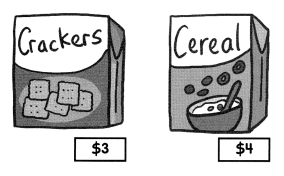Example
I want to buy 4 boxes of cereal.
I will have to give the cashier $16. Story __________________ Answer: I want to buy cracked which costs$3

I want to buy cereal which costs \$4.

Challenging Practice

Question 1.
Steve starts reading a book on page 7. He reads the book for 4 days. He reads 3 pages each day. Which page will Steve stop at on the 4th day?

Given,

Steve starts reading a book on page 7.

He reads the book for 4 days.

He reads 3 pages each day.

So, 4 x 3 = 1212 + 7 = 19

Therefore, on the 4th day, steve will stop on the 19th page.

Question 2.
The music teacher is selecting children to sit in the front row at a concert. 100 children are given numbers 1 to 100. The teacher first picks the child with the number 3. He then skip-counts by tens to pick the other children. What are the numbers of the other children who are picked?
The numbers are ___________________

Given,

The music teacher is selecting children to sit in the front row at a concert.

100 children are given numbers 1 to 100.

The teacher first picks the child with the number 3.

He then skip-counts by tens to pick the other children.

Problem Solving

Solve the riddle.

Question 1.
I am a two-digit number. I am more than 20 but less than 30. I can be found in both the multiplication tables of 3 and 4. What number am I?

Given,

I am a two-digit number.

I am more than 20 but less than 30.

I can be found in both the multiplication tables of 3 and 4.

Let the number be a

20 < a > 30

the numbers that are both multiple of 3 and 4 and between 20 and 30 is are 24 only ( 3 x 8 = 4 x 6 )

Therefore, the number is 24.

Chapter Review/Test

Vocabulary

Fill in the blanks with words from the box.

skip-counting
dot paper
related multiplication facts

Question 1.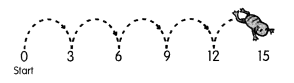____________ is fun!

Question 2.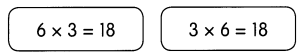are examples of _____________.

Concepts and Skills

Skip count to find the missing numbers.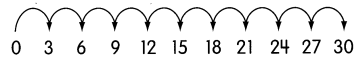Question 3.
9 × 3 = ____________

Question 4.
___________ × 3 = 24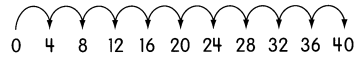Question 5.
_________ × 4 = 16

Question 6.
_________ × 4 = 36

Find the missing numbers.

Question 7.
8 groups of 3 = __________ × 3
= ___________

Question 8.
7 groups of 4 = ___________ × 4
= ___________

Use dot paper to find the missing numbers.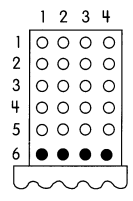Question 9.
6 × 4 = 5 groups of 4 + ___________ group of 4
= ___________ + 4
= ___________

Problem Solving

Use skip-counting or dot paper to solve.

Question 10.
Caleb ties sets of 3 medals with a piece of ribbon. He ties 10 sets of medals. How many medals does Caleb have in all?